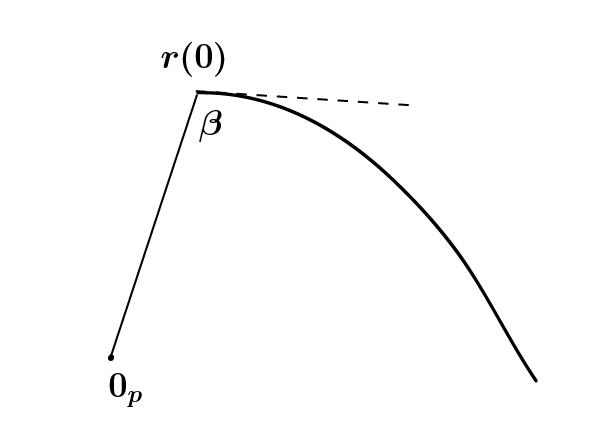I am now aiming to prove an important fixed point theorem:

Theorem 1 (Elie Cartan) Let${K}$ be a compact Lie group acting by isometries on a simply connected, complete Riemannian manifold${M}$ of negative curvature. Then there is a common fixed point of all${k \in K}$.

There are several ingredients in the proof of this result. These will provide examples of the techniques that I have discussed in past posts.

Geodesic triangles

Let${M}$ be a manifold of negative curvature, and let${V}$ be a normal neighborhood of${p \in M}$; this means that${\exp_p}$ is a diffeomorphism of some neighborhood of${0 \in T_p(M)}$ onto${V}$, and any two points in${V}$ are connected by a unique geodesic. (This always exists by the normal neighborhood theorem, which I never proved. However, in the case of Cartan’s fixed point theorem, we can take${V=M}$ by Cartan-Hadamard.)

So take${a=p,b,c\in V}$. Draw the geodesics${\gamma_{ab}, \gamma_{ac}, \gamma_{bc}}$ between the respective pairs of points, and let${\Gamma_{ab}, \Gamma_{bc}}$ be the inverse images in${T_p(M) = T_a(M)}$ under${\exp_p}$. Note that${\Gamma_{ab}, \Gamma_{ac}}$ are straight lines, but${\Gamma_{bc}}$ is not in general. Let${a',b',c'}$ be the points in${T_p(M)}$ corresponding to${a,b,c}$ respectively. Let${A}$ be the angle between${\gamma_{ab}, \gamma_{ac}}$; it is equivalently the angle at the origin between the lines${\Gamma_{ab}, \Gamma_{ac}}$, which is measured through the inner product structure.

Now${d(a,b) = l(\gamma_{ab}) = l(\Gamma_{ab}) = d(a',b')}$ from the figure and since geodesics travel at unit speed, and similarly for${d(a,c)}$. Moreover, we have${d(b,c) = l(\gamma_{bc}) \geq l(\Gamma_{bc}) \leq d(b',c')}$, where the first inequality comes from the fact that${M}$ has negative curvature and${\exp_p}$ then increases the lengths of curves; this was established in the proof of the Cartan-Hadamard theorem.

We have evidently by the left-hand-side of the figure$\displaystyle d(b',c')^2 = d(a',c')^2 + d(a',b')^2 - 2d(a',b')d(a',c') \cos A.$

In particular, all this yields$\displaystyle \boxed{d(b,c)^2 \geq d(a,c)^2 + d(a,b)^2 - 2d(a,b)d(a,c) \cos A.}$

So we have a cosine inequality.

There is in fact an ordinary plane triangle with sides${d(a,b), d(b,c),d(a,c)}$, since these satisfy the appropriate inequalities (unless${a,b,c}$ lie on the same geodesic, which case we exclude). The angles${A',B',C'}$ of this plane triangle satisfy$\displaystyle A \leq A'$

by the boxed equality. In particular, if we let${B}$ (resp.${C}$) be the angles between the geodesics${\gamma_{ab}, \gamma_{bc}}$ (resp.${\gamma_{ac}, \gamma_{bc}}$), then by symmetry and${A'+B'+C=\pi}$$\displaystyle \boxed{A + B+ C \leq \pi.}$

This is a fact which I vaguely recall from popular-math books many years back.   The rest is below the fold.

Derivative of the distance function

The next ingredient for the proof of Cartan’s fixed point theorem is the derivative of the distance function as one point varies along a curve.

Henceforth I will assume that${M}$ is simply connected, complete, and of negative curvature.

Let${q}$ be a curve in${M}$, parametrized by arc length, not containing the point${p \in M}$. Let${F_q(t) = d(p,q(t))}$.

Proposition 2 We have$\displaystyle \boxed{ F'(0) = - \cos \alpha }$

for${\alpha}$ the angle between the curve${q}$ and the unique geodesic${\gamma}$ from${p}$ to${q(0)}$.First of all, it’s enough to compute${\frac{d}{dt}(F^2)_{t=0}}$; this will fit in better with the law of cosines.

Now${\exp_p: T_p(M) \rightarrow M}$ is a diffeomorphism. So we can consider the curve${r}$ in${T_p(M)}$ corresponding to${q}$. By Hopf-Rinow, we have$\displaystyle d(p,q(t)) = d( 0_p, r(t)) = |r(t)|$

where the latter distance is in${T_p(M)}$.

Next, we compute$\displaystyle |r(t)|^2 - |r(0)|^2 = |r(t)-r(0)|^2 - 2 |r(0)||r(t) - r(0)| \cos \beta_t,$

as shown in the figure.As${t \rightarrow 0}$, the first term${|r(t)-r(0)|^2}$ is${O(t^2)}$ and contributes nothing to the derivative. Now${|r(t) - r(0)|}$ is approximately${t |r'(0)|}$ for${t}$ small, so putting this together we get$\displaystyle \frac{d}{dt} d(p,q(t))^2 |_{t=0} = -2d(p,q(0)) |r'(0)| \cos \beta,$

as in the figureand therefore$\displaystyle F'(0) = - |r'(0)| \cos \beta.$

Now${ |r'(0)| \cos \beta}$ is the length of the projection of${r'(0)}$ onto the line from${0}$ to${r(0)}$. Let the components be${A,B}$, where${A}$ is the component in the direction of${0}$ to${r(0)}$, and${B}$ is orthogonal to it, so${r'(0)=A+B}$ and${|r'(0)| \cos \beta = |A|}$. In the curve${q(t) = \exp_p(r(t))}$, we have${A'=\exp_p(A) \in T_{q(0)}(M)}$ orthogonal to${B'=\exp_p(B)}$ and${\exp_p}$ in the direction of the geodesic${\gamma}$. Also,${|A'| = |A|}$; straight lines through the origin get sent to geodesics in${M}$, which travel at constant speed.

Note that${|A'+B'|=1}$ by the assumption on${q}$.

In particular,${\cos \alpha}$ is the length${|A'|}$—which we have just shown is${|A| = -|r'(0)|\cos \beta}$.

I have followed Helgason here.